Note: The other languages of the website are Google-translated. Back to English

## Count number of cells between two values / dates in Excel

Have you ever tried to get or count the number of cells between two given numbers or dates in Excel as below screenshot shown? This article will talk about some useful formulas for dealing with it.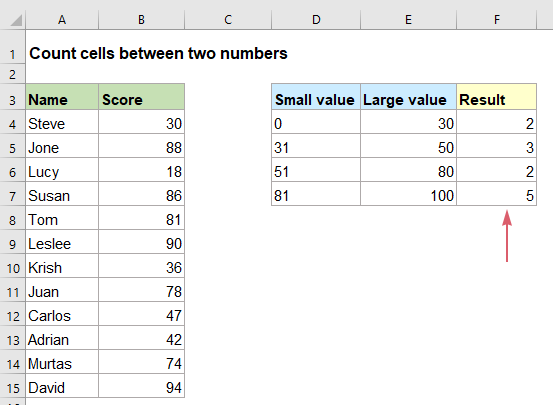#### Count the number of cells between two values

In Excel, the COUNTIFS function can help you to get the number of cells between two numbers. The generic syntax is:

=COUNTIFS(range,">="&small value cell,range,"<="&large value cell)
• range: The column range that contains the values you want to count;
• small value cell: The cell that contains the small value you want to use;
• large value cell: The cell that contains the large value you want to use;
• &: This separator is used to concatenate the cell value with the conditions.

1. Please enter or copy the below formula into a blank cell:

=COUNTIFS(\$B\$4:\$B\$15,">="&D2,\$B\$4:\$B\$15,"<="&E2)

2. Then, drag this formula to other cells that you want to apply this formula, and now, you will get the number of cells between the given numbers, see screenshot: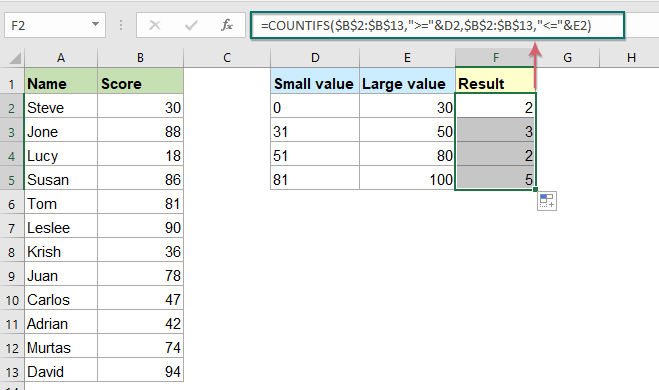Tips: You can also put the numbers into the formula directly instead of the cell references, for example, to get the number of cells between 30 and 80, please apply the below formula:

=COUNTIFS(\$B\$2:\$B\$13,">=30",\$B\$2:\$B\$13,"<=80")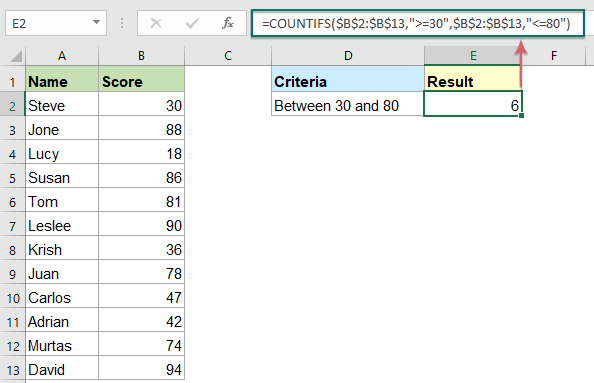#### Count the number of cells between two dates

This COUNTIFS function also can help to count the number of dates that fall between two specific dates. The generic syntax is:

=COUNTIFS(range ,">=" &start_date cell, range, "<=" &end_date cell)
• range: The column range that contains the date list;
• start_date cell: The cell that contains the start date you want to use;
• end_date cell: The cell that contains the end date you want to use;
• &: This separator is used to concatenate the date cell with the conditions.

1. Enter or copy the following formula into a blank cell where you want to put the result:

=COUNTIFS(\$B\$2:\$B\$13,">=" &D2, \$B\$2:\$B\$13, "<=" &E2)

2. Then, drag this formula down to other cells that you want to use this formula, and the number of cells between two specific dates have been calculated at once, see screenshot: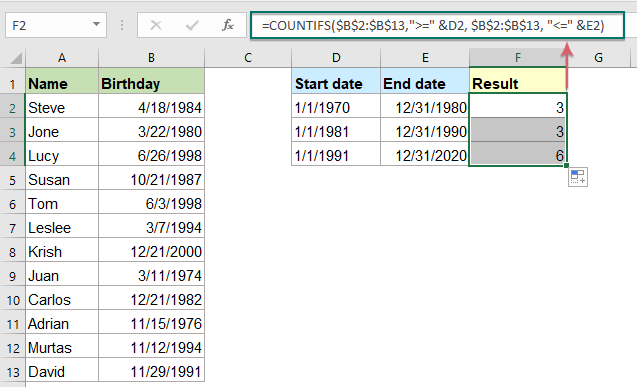Tips: Besides, you can type the start and end dates into the formula directly instead of using cell references. For example, to get the number of cells between 1/1/1985 and 1/1/2000, please apply the below formula:

=COUNTIFS(\$B\$2:\$B\$13,">=1/1/1985",\$B\$2:\$B\$13,"<=1/1/2000")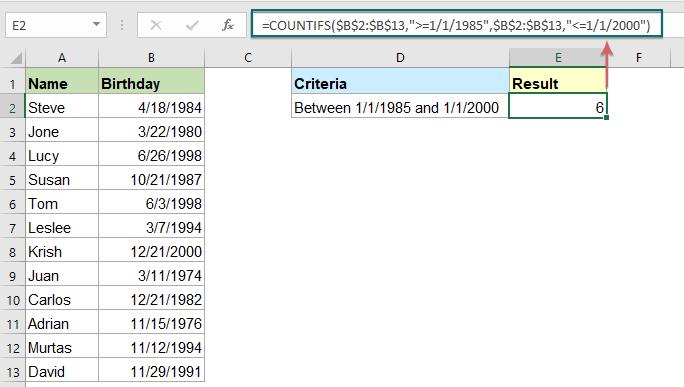#### Relative functions used:

• COUNTIFS:
• The COUNTIFS function returns the number of cells that meet one single criterion or multiple criteria.

#### More articles:

• Count number of cells not between two given numbers in Excel
• To count the number of cells between two numbers is a common task for us in Excel, but, in certain case, you may want to count the cells not between two given numbers. For example, I have a product list with the sales from Monday to Sunday, now, I need to get the number of cells which not between the specific low and high numbers. This article, I will introduce some formulas for dealing with this task in Excel.
• Count number of cells equals to one of many values in Excel
• Supposing, I have a list of products in column A, now, I want to get the total number of specific products Apple, Grape and Lemon which listed in range C4:C6 from column A as below screenshot shown. Normally, in Excel, the simple COUNTIF and COUNTIFS functions will not work in this scenario. This article, I will talk about how to solve this job quickly and easily with the combination of SUMPRODUCT and COUNTIF functions.
• Count number of cells not equal to many values in Excel
• In Excel, you may easily get the number of cells not equal to a specific value by using the COUNTIF function, but have you ever tried to count the cells which are not equal to many values? For example, I want to get the total number of the products in column A but exclude the specific items in C4:C6 as below screenshot shown. This article, I will introduce some formulas for solving this job in Excel.

### The Best Office Productivity Tools

#### Kutools for Excel - Helps You To Stand Out From Crowd

Would you like to complete your daily work quickly and perfectly? Kutools for Excel brings 300 powerful advanced features (Combine workbooks, sum by color, split cell contents, convert date, and so on...) and save 80% time for you.

• Designed for 1500 work scenarios, helps you solve 80% Excel problems.
• Reduce thousands of keyboard and mouse clicks every day, relieve your tired eyes and hands.
• Become an Excel expert in 3 minutes. No longer need to remember any painful formulas and VBA codes.
• 30-day unlimited free trial. 60-day money back guarantee. Free upgrade and support for 2 years.#### Office Tab - Enable Tabbed Reading and Editing in Microsoft Office (include Excel)

• One second to switch between dozens of open documents!
• Reduce hundreds of mouse clicks for you every day, say goodbye to mouse hand.
• Increases your productivity by 50% when viewing and editing multiple documents.
• Brings Efficient Tabs to Office (include Excel), Just Like Chrome, Firefox, And New Internet Explorer.